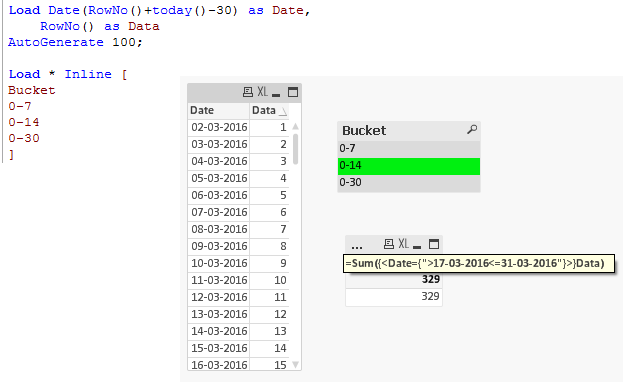# QlikView App Dev

Discussion Board for collaboration related to QlikView App Development.

Announcements
Become an analytics expert with Qlik's new 15 week course: Applied Data Analytics using Qlik Sense. READ MORE
cancel
Showing results for
Did you mean:Creator

## Creation of ranges

Hi,

I want to create a bucket where the range of bucket will be

'last 7 days' would be '0-7',

'last 14 days' would be '0-14' and 'last 30 days' would be '0-30'

this buckets should give me results of the previous 7, 14 , 30 days with reference to the present day..

i.e. for eg:- today is 31st March if i select on last 7 days bucket it should show me details from 25th march to 31st march, likewise for the rest of them..

Can anyone help me to achieve this..?

Regards,

Saniya.

1 Solution

Accepted SolutionsMVP

Bucket

0-7

0-14

0-30 ];

Now in you chart write the below expression. Make sure that your Date field is in proper date format

if(Bucket='0-7', sum({<Date = {">=\$(=date(max(Date)-7)<=\$(=max(Date))"}>}Amount),

if(Bucket='0-14', sum({<Date = {">=\$(=date(max(Date)-14)<=\$(=max(Date))"}>}Amount),

f(Bucket='0-30', sum({<Date = {">=\$(=date(max(Date)-30)<=\$(=max(Date))"}>}Amount))))

6 RepliesMVP

May be create flags in your script to do this.Champion III

hi,

AgingWeekSort:

days,Value

1 Week,1

2 Weeks, 2

> 2 Weeks,3

]

DaysSort:

[DayBands, Value1

1 Day, 1

2 Days, 2

3 Days, 3

> 3 Days, 4

]

u can create a map table like abve  and u will get  fileds in frontendMVP

Bucket

0-7

0-14

0-30 ];

Now in you chart write the below expression. Make sure that your Date field is in proper date format

if(Bucket='0-7', sum({<Date = {">=\$(=date(max(Date)-7)<=\$(=max(Date))"}>}Amount),

if(Bucket='0-14', sum({<Date = {">=\$(=date(max(Date)-14)<=\$(=max(Date))"}>}Amount),

f(Bucket='0-30', sum({<Date = {">=\$(=date(max(Date)-30)<=\$(=max(Date))"}>}Amount))))Champion III

orelse

if(urfield <= 7, '7 Day', if(urfield ='0-14 , '14Days', if(urfield = 30,'30days' ,'31days')))) as DaySMVP

One way could be creating bucket as island and trying expression like:

=Sum({<Date={">\$(=Date(Today()-SubField(Bucket,'-',2)))<=\$(=Today())"}>}Data)Creator
Author

Hi Kush,

Thanks for the prompt revert.. It was quite helpful..

Regards,

Saniya ShaikhCommunity Browser